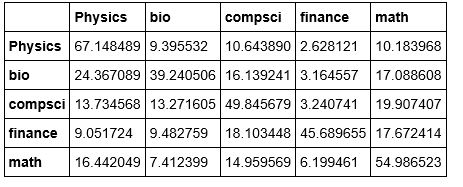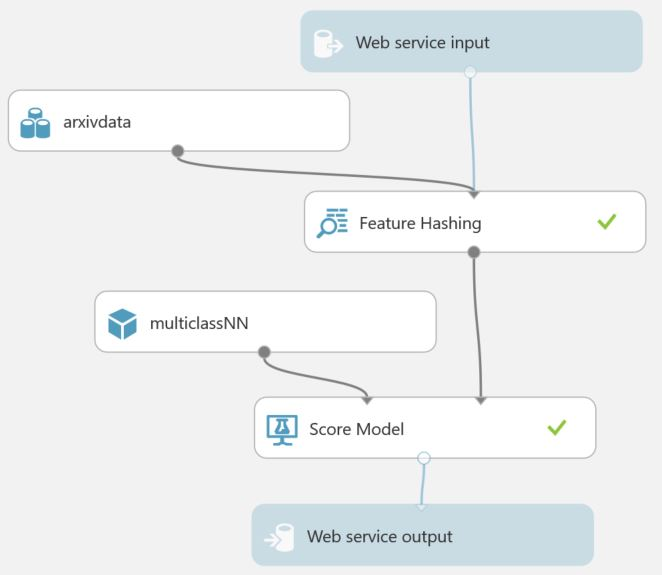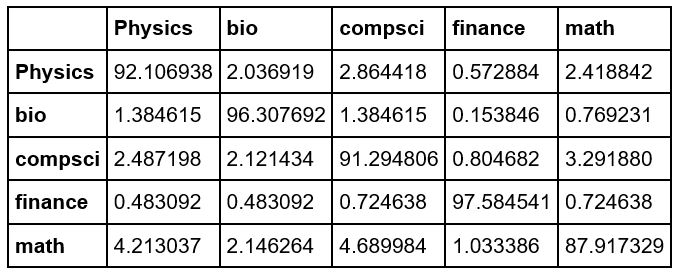# Cloud SaaS: Machine Learning with Azure ML

The large public cloud vendors including Amazon, Microsoft and Google have invested heavily to provide Infrastructure as a Service (IaaS) to their customers.   The result of this intense competition has been a race to the bottom for pricing of the basic compute and storage services.   Great news for the customers.  We are also beginning to see a similar convergence around Platform as a Service as more and more basic tooling for building cloud apps becomes standard.   Clearly each vendor has some unique capabilities, but the real differentiation between vendors is taking place at the level of cloud software-as-a-service (SaaS) offerings.     The most interesting areas for science relevant services are machine learning, stream analytics and big data analysis tools.    Each of the three big cloud vendors have offering in this space and so do others like IBM, Salesforce and Cloudera.   The next few posts will be about some experience using these tools.   I will start with AzureML because I have access to it.

I decided to  redo some of my streaming scientific text analysis projects (described earlier: part 1 and part 2) using Microsoft’s new AzureML.   I’ll return to the full streaming topic using Azure stream analytics in the next post.

If you are not familiar with AzureML, the machine learning toolkit for Microsoft Azure, you should give it a try.   In fact, you can try it for free.  Go to https://studio.azureml.net.   AzureML is based on a “drop-and-drag” component composition model where you can build a solution to a machine learning problem by dragging parts of the solution from a pallet of tools.   This post is not intended as a tutorial for AzureML. There are tons of good tutorials on line.    I would start with the ones on the studio.azureml.net home page.     This post is a description of what I was able to do with AzureML for the basic task of classifying scientific documents.  More specifically, we have a collection of RSS feed documents that describe new scientific results and research papers from various sources, but the best stuff for our purposes comes from the Cornell University Library ArXiv RSS feed.   Each item in the collection is a tuple consisting of the article title, the abstract and a classification into one of the basic science disciplines including Physics, Mathematics, Computer Science, Biology and Finance.   Here is a sample.
``` ['A Fast Direct Sampling Algorithm for Equilateral Closed Polygons. (arXiv:1510.02466v1 [cond-mat.stat-mech])', 'Sampling equilateral closed polygons is of interest in the statistical study of ring polymers. Over the past 30 years, previous authors have proposed a variety of simple Markov chain algorithms (but have not been able to show that they converge to the correct probability distribution) and complicated direct samplers (which require extended-precision arithmetic to evaluate numerically unstable polynomials). We present a simple direct sampler which is fast and numerically stable. ', 'Physics'] ```
The challenge is to use only the abstract to predict the classification.  (As you can see from this example a reasonable guess might be Physics, Math or Computer Science, so it is not that easy.)

A typical AzureML solution looks like the one below that has been configured to train a neural network to classify the items in our list of science abstracts.  It is easy to mistake this diagram for a data flow graph but it is really a workflow dependency diagram represented as a directed acyclic graph.   Each arrow represents a dependency of the output of one task as part of the input of the next.   Each box represents one of the analysis subtasks.   When you run the training experiment the subtasks that complete have a green checkmark.   It is possible to inspect the result of any subtask by clicking on the tail of the result arrow.  Doing this presents you with several possible choices that include saving the result, visualizing it in tabular form or, in some some cases, viewing it in a IPython (Jupyter) notebook.Figure  1.  AzureML Studio workflow diagram for the Multiclass Neural network and the Python module for creating the studio version of the arxivdata data set.

To understand this workflow, it is best to start at the top which is where the data source comes into the picture.     In the case here we are going to take the data from a Azure blob storage public archive http://esciencegroup.blob.core.windows.net/scimlpublic.   The dataset is sciml_data_arxiv.p which is a Python pickle file.   A recent addition to AzureML that I was very pleased to see was the introduction of a way to build a new component from R or Python.  Hence it was easy to write a small Python preprocessing file that could read the data and clean it up a bit and present it to the rest of the AzureML studio.   The data interface between Python and AzureML is based on Pandas data frames.  The output of the Python module can be accessed on the output labeled 1.   We could have put this directly into the next stage in our workflow, but we can also save it to AzureML studio.   We have done that in this case and we used a copy of that dataset as the box “arxivdata”.    The data set has three columns and each row represents one document and it is a triple (classification, the abstract of the documents, the title of the document).   As we move through the workflow we will add columns and, for various tasks, we restrict attention to only a few columns

The second box down is “Feature Hashing”.    This box builds a vectorizer based on the vocabulary in the document collection.   This version comes from the Vowpal Wabbit library and its role is to convert each document into a numerical vector corresponding to the key words and phrases in the document collection.    This numeric representation is essential for the actual machine learning phase.   To create the vector, we tell the feature hasher to only look at the document text.   What happens on the output is that the vector of numeric values for the abstract text is now appended to the tuple for each document.   Our table now has a very large number of columns: class, the document text, the title,  vector, … vector[n] where n is the number of “features”

In the next box “Split Data” we split the resulting table into a training set and a test set.   In this case we have configured the Split Data box to put 75% into the training set and the remainder in the test set.

For the machine learning we need to select a machine learning algorithm,  and some columns of the data to use for the training.  To select the columns for the training we use a “project columns” task and we select the “class” and the feature vector components.  (we don’t need the document text or title.)  AzureML has a reasonably large number of the standard machine learning modules.   We found three that were good but by a small margin “Multiclass Neural Network” was the best performer.   Each machine learning module has various parameters that can be selected to tune the method.   For all the experiments described here, we just used the default parameter settings[i].   The “Train Model” component accepts as one input a binding to one of the ML methods (recall this is not a dataflow graph) and the other input is the projected training data.    The output of the Train Model task is not data per se but a trained model that may also be saved for later use.    This trained model can now be used to classify our test data and that is what we do with the “Score Model” component.   The score model appends another new column to our table called Scored Label which is the classification predicted by the trained model for that each row.

Finally can see how we did by using the “Evaluate Model” component which computes a confusion matrix.   Each row of the matrix tells up how the documents in that class were classified.   In this experiment the confusion matrix is shown in Figure 2 below.Figure 2.  Confusion matrix for the ArXiv data set which includes some duplicate of bio and math documents.

There are several points worth noting here.   First bio and finance documents were recognized with high accuracy.   This is somewhat artificial because documents in those category were each repeated twice in the original data.   Hence after the splitting (by ¾ test, ¼ training)  a large fraction of the test set for these documents (about 75%) will be in both the training set and the test set hence they are easily recognized.  We have a more recent collection of ArXiv documents which do not include any of the training set items.  Figure 3 below shows the confusion matrix for this case.  It is clear than the classifier had a hard time distinguishing Physics, Math and Computer Science.    We have no doubt that we could achieve better results if fine-tuned the neural net parameters.Figure 3.   Confusion matrix for the multiclass neural network classifier using the more recent ArXiv data

We will show a better classifier in the second half of this article.

[i] By not tuning the parameters of each ML algorithm we are doing them an injustice.  But it takes expert knowledge of what the algorithm does and lots of time to experiment to find the right settings.   I was surprised at how well the default parameters worked.

## Creating a web service from our trained classifier

One of the most impressive features of AzureML is how easily it can convert a trained model like the one above into a function web service.    In fact, it is very cool.   One click on the pallet button for creating a web service transform the diagram in Figure 1 to the diagram in Figure 4.Figure 4.   A web service that was automatically created from the experiment in Figure 1.

We can test this webservice from the studio or we can go ahead and deploy it to the web.   Once it has been deployed AzureML will even generate the C# or Python or R code you can use to deploy it.  In this case the code for Python is

```import urllib2
import json
data =  {
"Inputs": {
"input1":
{
"ColumnNames": ["class","document","title"],
"Values": [["value","value","value"], ["value","value","value"], ]
},        },
"GlobalParameters": {
}
}
body = str.encode(json.dumps(data))
url = 'https://ussouthcentral.services.azureml.net/
9888e0b134724d0c834f009574275f65/execute?api-version=2.0&details=true'
api_key = 'abc123' # Replace this with the API key for the web service
'Authorization':('Bearer '+ api_key)}
try:
response = urllib2.urlopen(req)
print(result)
except urllib2.HTTPError, error:
print("The request failed with status code: " + str(error.code))
print(error.info())
```

The input is defined by the data template where you can supply one or more of the arxiv tuples.   A copy of an IPyhon notebook that invokes the webservice and computes the confusion matrix is linked here.

## Creating a more interesting classifier.

The example so far does not fully illustrate the power of the AzureML studio.    I decided to try to build a classifier that uses three different machine learning algorithms all trained on the same data.   Then I would use a majority vote to select the wining choice.   I have argued in previous posts that picking a single choice for this science data is not a good idea because science is very multidisciplinary.   The example sited above illustrates this point.   So I trained two additional ML algorithms: a boosted decision tree and a two class support vector machine (converted to a multiclass algorithm using a “one vs all multiclass” module I found in the studio pallet.  I saved the trained models for each.   Then I started with the diagram in Figure 4 and I started adding things.   The result is in Figure 5Figure 5.   A best of three classifier service created by modifying the service in Figure 4.

You will notice that this is almost like three copies of the service in Figure 4.  The difference is that I needed to reduce the output to a smaller set of tuples to give to a simple python module to do the majority voting.   This was done with column projections.   The leftmost project selects the “class” and “Scored Labels” columns (discarding the title document and the doc vector)  the second selects “class” and “Scored Labels” and the third selects only the “Scored Labels”.    Then using an “Add Column” component we append the last column to the output of the second project.   By doing this we now have two inputs to a Python Script module (which is limited to two dependences).    The Python code inside the script module is shown below.   In this version we assume that if all three scored labels disagree we should pick the first (from the multiclassNN classifier) as the main one and, arbitrarily pick the second scored Label as the “second choice”.   Otherwise if any two agree that becomes the first choice.

```#   Param: a pandas.DataFrame
#   Param: a pandas.DataFrame
def azureml_main(dataframe1 = None, dataframe2 = None):
tclass = dataframe1["class"]
scored1 = dataframe1["Scored Labels"]
scored2 = dataframe2["Scored Labels"]
scored3 = dataframe2["Scored Labels (2)"]
scored = []
second = []
lclass = []
for i in range(0, len(tclass)):
lclass.extend([tclass[i]])
if scored2[i] == scored3[i]:
scored.extend([scored2[i]])
second.extend([scored1[i]])
else:
scored.extend([scored1[i]])
second.extend([scored2[i]])
data = {'class': lclass, 'Scored Labels': scored, 'second': second}
df = pd.DataFrame(data, columns=['class', 'Scored Labels', 'second'])

# Return value must be of a sequence of pandas.DataFrame
return df,
```

The webservice now returns three values.   The original class designation from ArXiv, our best-of-three choice and a second choice (which may be the same of best-of-three).    If we now look at the confusion matrix for the original arxivdata data (including the training examples) we getFigure 6.   Best-of-three with original data.

Figure 7 shows the result when we use the dataset arxiv-11-1-15 that contains no documents from the training.Figure 7.  Best of three with arxiv-11-1-15 data.

The improvement over the original method shown in Figure 3 is about 15%.   Of course we are now giving the classifier two chances to get the answer right.

As mentioned above we probably could do much better by tuning the ML parameters.   But the point of this post was to show you what is possible with a very modest effort with AzureML.    In the next post we will look at performance issues, scalability and streaming examples.